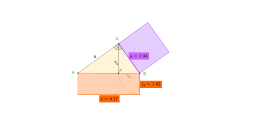# Hypotenuse 72524

We know the height of the hypotenuse h = 4cm and the hypotenuse c = 19cm in a right triangle. How to calculate the segments of legs - sections on the hypotenuse c1, c2

c1 =  18.1168 cm
c2 =  0.8832 cm

### Step-by-step explanation:

Our quadratic equation calculator calculates it.

Try calculation via our triangle calculator.Did you find an error or inaccuracy? Feel free to write us. Thank you!

Tips for related online calculators
Are you looking for help with calculating roots of a quadratic equation?
Do you have a linear equation or system of equations and looking for its solution? Or do you have a quadratic equation?
The Pythagorean theorem is the base for the right triangle calculator.
Do you want to convert time units like minutes to seconds?

#### Grade of the word problem:

We encourage you to watch this tutorial video on this math problem: